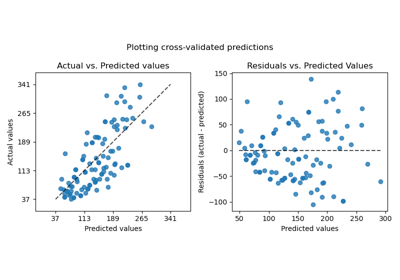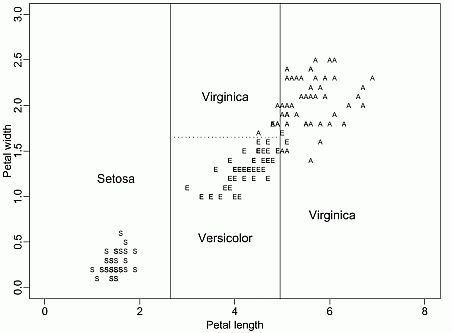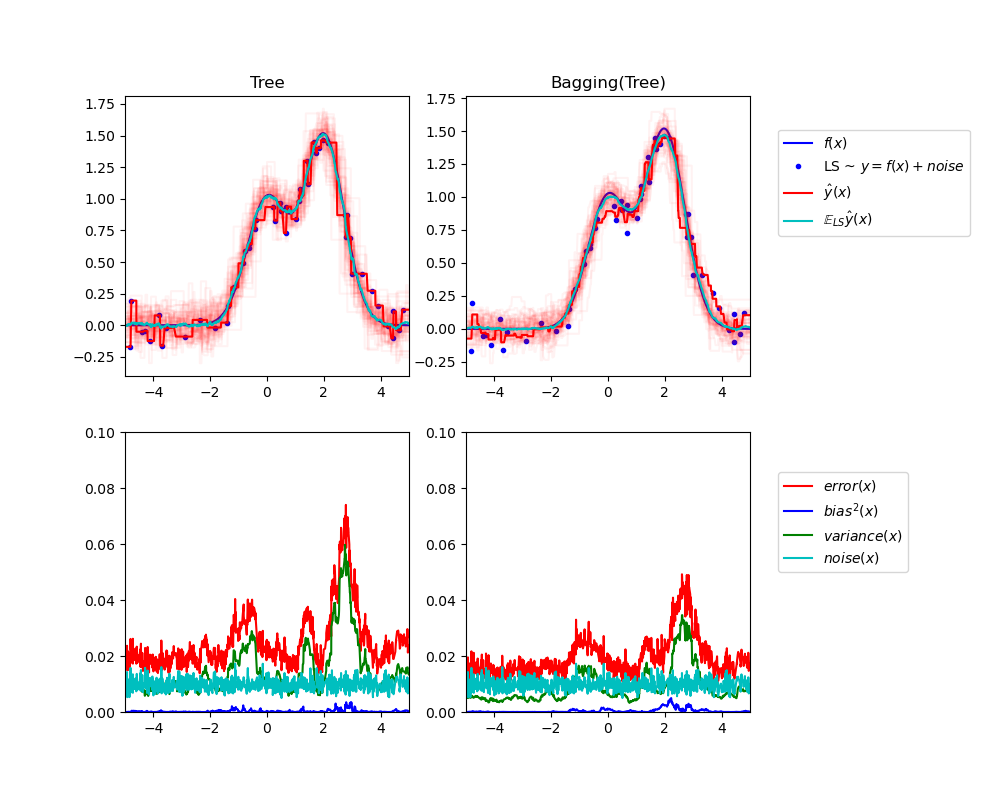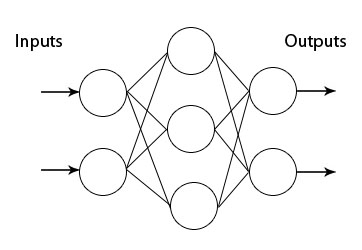Need help with ml-interview?
Click the “chat” button below for chat support from the developer who created it, or find similar developers for support.ShuaiW657 Stars209 Forks19 Commits1 Opened issuesDescription

Preparing for machine learning interviews!
?

Need anything else?Contributors list# 14,880
Python
Jupyter...
pandas
seaborn
13 commits

This repo is deprecated. Please go to the new repo, Data Science Question Answer

This repository covers how to prepare for machine learning interviews, mainly in the format of questions & answers. Asides from machine learning knowledge, other crucial aspects include:

Go directly to machine learning

Your resume should specify interesting ML projects you got involved in the past, and quantitatively show your contribution. Consider the following comparison:

Trained a machine learning system

vs.

Trained a deep vision system (SqueezeNet) that has 1/30 model size, 1/3 training time, 1/5 inference time, and 2x faster convergence compared with traditional ConvNet (e.g., ResNet)

We all can tell which one is gonna catch interviewer's eyeballs and better show case your ability.

In the interview, be sure to explain what you've done well. Spend some time going over your resume before the interview.

SQL

Although you don't have to be a SQL expert for most machine learning positions, the interviews might ask you some SQL related questions so it helps to refresh your memory beforehand. Some good SQL resources are:

Machine learning

First, it's always a good idea to review Chapter 5 of the deep learning book, which covers machine learning basics.

Linear regression

• how to learn the parameter: minimize the cost function
• how to minimize cost function: gradient descent
• regularization:
• L1 (lasso): can shrink certain coef to zero, thus performing feature selection
• L2 (ridge): shrink all coef with the same proportion; almost always outperforms L1
• combined (Elastic Net):
• assumes linear relationship between features and the labelLogistic regression

• Generalized linear model (GLM) for classification problems
• Apply the sigmoid function to the output of linear models, squeezing the target to range [0, 1]
• Threshold to make prediction: if the output > .5, prediction 1; otherwise prediction 0
• a special case of softmax function, which deals with multi-class problem

KNN

Given a data point, we compute the K nearest data points (neighbors) using certain distance metric (e.g., Euclidean metric). For classification, we take the majority label of neighbors; for regression, we take the mean of the label values.

Note for KNN technically we don't need to train a model, we simply compute during inference time. This can be computationally expensive since each of the test example need to be compared with every training example to see how close they are.

There are approximation methods can have faster inference time by partitioning the training data into regions.

Note when K equals 1 or other small number the model is prone to overfitting (high variance), while when K equals number of data points or other large number the model is prone to underfitting (high bias)SVM

• can perform linear, nonlinear, or outlier detection (unsupervised)
• large margin classifier: not only have a decision boundary, but want the boundary to be as far from the closest training point as possible
• the closest training examples are called the support vectors, since they are the points based on which the decision boundary is drawn
• SVMs are sensitive to feature scalingDecision tree

• Non-parametric, supervised learning algorithms
• Given the training data, a decision tree algorithm divides the feature space into regions. For inference, we first see which region does the test data point fall in, and take the mean label values (regression) or the majority label value (classification).
• Construction: top-down, chooses a variable to split the data such that the target variables within each region are as homogeneous as possible. Two common metrics: gini impurity or information gain, won't matter much in practice.
• Advantage: simply to understand & interpret, mirrors human decision making
• can overfit easily (and generalize poorly)if we don't limit the depth of the tree
• can be non-robust: A small change in the training data can lead to a totally different tree
• instability: sensitive to training set rotation due to its orthogonal decision boundariesBagging

To address overfitting, we can use an ensemble method called bagging (bootstrap aggregating), which reduces the variance of the meta learning algorithm. Bagging can be applied to decision tree or other algorithms.

Here is a great illustration of a single estimator vs. bagging• Bagging is when samlping is performed with replacement. When sampling is performed without replacement, it's called pasting.
• Bagging is popular due to its boost for performance, but also due to that individual learners can be trained in parallel and scale well
• Ensemble methods work best when the learners are as independent from one another as possible
• Voting: soft voting (predict probability and average over all individual learners) often works better than hard voting
• out-of-bag instances (37%) can act validation set for bagging

Random forest

Random forest improves bagging further by adding some randomness. In random forest, only a subset of features are selected at random to construct a tree (while often not subsample instances). The benefit is that random forest decorrelates the trees.

For example, suppose we have a dataset. There is one very predicative feature, and a couple of moderately predicative features. In bagging trees, most of the trees will use this very predicative feature in the top split, and therefore making most of the trees look similar, and highly correlated. Averaging many highly correlated results won't lead to a large reduction in variance compared with uncorrelated results. In random forest for each split we only consider a subset of the features and therefore reduce the variance even further by introducing more uncorrelated trees.

I wrote a notebook to illustrate this point.

In practice, tuning random forest entails having a large number of trees (the more the better, but always consider computation constraint). Also,

min_samples_leaf
(The minimum number of samples at the leaf node)to control the tree size and overfitting. Always CV the parameters.

Feature importance

In a decision tree, important features are likely to appear closer to the root of the tree. We can get a feature's importance for random forest by computing the averaging depth at which it appears across all trees in the forest.

Boosting

How it works

Boosting builds on weak learners, and in an iterative fashion. In each iteration, a new learner is added, while all existing learners are kept unchanged. All learners are weighted based on their performance (e.g., accuracy), and after a weak learner is added, the data are re-weighted: examples that are misclassified gain more weights, while examples that are correctly classified lose weights. Thus, future weak learners focus more on examples that previous weak learners misclassified.

Difference from random forest (RF)

• RF grows trees in parallel, while Boosting is sequential
• RF reduces variance, while Boosting reduces errors by reducing bias

XGBoost uses a more regularized model formalization to control overfitting, which gives it better performance

Stacking

• Instead of using trivial functions (such as hard voting) to aggregate the predictions from individual learners, train a model to perform this aggregation
• First split the training set into two subsets: the first subset is used to train the learners in the first layer
• Next the first layer learners are used to make predictions (meta features) on the second subset, and those predictions are used to train another models (to obtain the weigts of different learners) in the second layer
• We can train multiple models in the second layer, but this entails subsetting the original dataset into 3 partsMLP

A feedforward neural network where we have multiple layers. In each layer we can have multiple neurons, and each of the neuron in the next layer is a linear/nonlinear combination of the all the neurons in the previous layer. In order to train the network we back propagate the errors layer by layer. In theory MLP can approximate any functions.CNN

The Conv layer is the building block of a Convolutional Network. The Conv layer consists of a set of learnable filters (such as 5 * 5 * 3, width * height * depth). During the forward pass, we slide (or more precisely, convolve) the filter across the input and compute the dot product. Learning again happens when the network back propagate the error layer by layer.

Initial layers capture low-level features such as angle and edges, while later layers learn a combination of the low-level features and in the previous layers and can therefore represent higher level feature, such as shape and object parts.RNN and LSTM

RNN is another paradigm of neural network where we have difference layers of cells, and each cell only take as input the cell from the previous layer, but also the previous cell within the same layer. This gives RNN the power to model sequence.This seems great, but in practice RNN barely works due to exploding/vanishing gradient, which is cause by a series of multiplication of the same matrix. To solve this, we can use a variation of RNN, called long short-term memory (LSTM), which is capable of learning long-term dependencies.

The math behind LSTM can be pretty complicated, but intuitively LSTM introduce - input gate - output gate - forget gate - memory cell (internal state)

LSTM resembles human memory: it forgets old stuff (old internal state * forget gate) and learns from new input (input node * input gate)word2vec

• Shallow, two-layer neural networks that are trained to construct linguistic context of words
• Takes as input a large corpus, and produce a vector space, typically of several hundred dimension, and each word in the corpus is assigned a vector in the space
• The key idea is context: words that occur often in the same context should have same/opposite meanings.
• Two flavors
• continuous bag of words (CBOW): the model predicts the current word given a window of surrounding context words
• skip gram: predicts the surrounding context words using the current wordGenerative vs discriminative

• Discriminative algorithms model p(y|x; w), that is, given the dataset and learned parameter, what is the probability of y belonging to a specific class. A discriminative algorithm doesn't care about how the data was generated, it simply categorizes a given example
• Generative algorithms try to model p(x|y), that is, the distribution of features given that it belongs to a certain class. A generative algorithm models how the data was generated.

Given a training set, an algorithm like logistic regression or the perceptron algorithm (basically) tries to find a straight line—that is, a decision boundary—that separates the elephants and dogs. Then, to classify a new animal as either an elephant or a dog, it checks on which side of the decision boundary it falls, and makes its prediction accordingly.

Here’s a different approach. First, looking at elephants, we can build a model of what elephants look like. Then, looking at dogs, we can build a separate model of what dogs look like. Finally, to classify a new animal, we can match the new animal against the elephant model, and match it against the dog model, to see whether the new animal looks more like the elephants or more like the dogs we had seen in the training set.